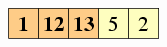# Chapter 20. Sorting

Sorting an array is one of the most fundamental problems in computer science. The basic problem is this: Suppose we have a list of numbers.We want to reorder the list so that the numbers appear in increasing order.Sorting is fundamental to computer science for several reasons: First, real programs often need to sort data; second, sorting techniques prove to be a foundation for many other computational problems; and finally, the problem is relatively simple to analyze, but still complex enough to have some interesting intricacies. Thus, it is a perfect showcase problem for study and research.

## 20.1. Selection sort

There are many reasonable ways to approach the problem of sorting an array. Most of the obvious ones are also relatively easy to analyze. We start with the selection sort algorithm.⇒We find the smallest element and swap it into the first position.⇒Then we find the smallest element right of the first position and swap it into the second position.⇒Then we find the smallest element right of the second position and swap it into the third position.⇒We continue doing this, each time determining the proper number to place in the next position of the array, until we've completed the array.

In writing this as a program, we'll use a variable `i` to track which position we are currently trying to swap into. We'll have a loop that iterates `i` through each index of the array. For each value of `i`, we must determine where to swap from. To determine this, we use another loop, using a variable `j` that steps through every index above `i`; each time we find a smaller element, we change `min` to refer to that element's index. After going through the inner loop over `j`'s, then, `min` will hold the index of the smallest number in position `i` or beyond. This is the position that we swap with position `i`. Then we can proceed to the next `i`.

```public void selectionSort(int[] data) {     for(int i = 0; i < data.length; i++) {         int min = i;         for(int j = i + 1; j < data.length; j++) {             if(data[j] < data[min]) min = j;         }         int t = data[min];         data[min] = data[i];         data[i] = t;     } } ```

## 20.2. Insertion sort

Insertion sort is an alternative sorting algorithm. For it, we keep the first segment of the array sorted, and we slowly grow this segment, element by element, until it encompasses the entire array.Segment starts with first element only.Then we grow it to the first two elements.Then the first three elements.Then the first four elements.… until the segment covers the entire array.

Each time we want to expand the segment by an element, the only thing we need to do is to insert the new element into the already sorted list — hence the name insertion sort. The insertion process involves shifting the elements of the old segment that are greater than the new element up by one position, to make room for the new element's proper position.

To implement this in a program, we need a variable to keep track of how large the current segment is; we'll use `i` for this. Each iteration, our goal is to increment `i` by one, which requires us to insert element `i` into the first `i` elements. To shift elements upward to make room, we'll use another variable, `j`, which will start at `i` − 1 and move down until element `j` is less than element `i`.

```public void insertionSort(int[] data) {     for(int i = 1; i < data.length; i++) {         int t = data[i];         int j = i - 1;         while(j >= 0 && data[j] > t) {             data[j + 1] = data[j];             j--;         }         data[j + 1] = t;     } } ```

## 20.3. Mergesort

The selection sort and insertion sort algorithms, along with most other intuitive techniques, take O(n²) time. It turns out, though, that a different algorithm called Mergesort does better.

The Mergesort algorithm is based on the idea of recursion. In particular, given an array, we will first recursively sort the first and second halves of the array separately. Then we will merge the two halves together to attain our final result.

 1. We start with an array.2. Divide the array into two halves.and3. Recursively sort each half.and4. Merge the sorted halves.Implementing Mergesort, as done in Figure 20.1, is really not too complex. Our method will take two indices as parameters, `start` and `stop`, representing which segment of the array to be sorted; this is so that the recursive calls can specify which segment to sort. These indices represent the first index inside the segment, and the first index after the segment.

Figure 20.1: The Mergesort algorithm.

```public void mergeSort(int[] data) {     if(data.length <= 1) return;               // Base case: just 1 elt     int[] a = new int[data.length / 2];        // Split array into two     int[] b = new int[data.length - a.length]; //   halves, a and b     for(int i = 0; i < data.length; i++) {         if(i < a.length) a[i] = data[i];         else             b[i - a.length] = data[i];     }     mergeSort(a);                              // Recursively sort first     mergeSort(b);                              //   and second half.     int ai = 0;                                // Merge halves: ai, bi     int bi = 0;                                //   track position in     while(ai + bi < data.length) {             //   in each half.         if(bi >= b.length || (ai < a.length && a[ai] < b[bi])) {             data[ai + bi] = a[ai]; // (copy element of first array over)             ai++;         } else {             data[ai + bi] = b[bi]; // (copy element of second array over)             bi++;         }     } } ```

Dividing the array into two and recursively sorting each half is simply a matter of finding where to split the segment (index `mid`) and making the recursive calls. The bulk of the implementation is spent in the merging. Here, we use two indices, `i` and `j`, referring to how far we've merged elements from the two half-segments. We copy elements from the half-segments into another array, each time incrementing `i` or `j`, until the other array is filled. Finally, we copy all the elements from the merged array back into the original.